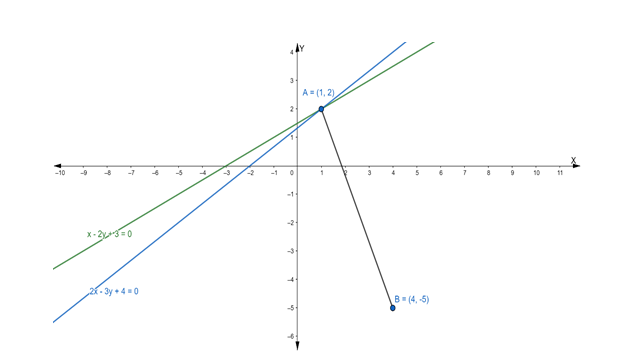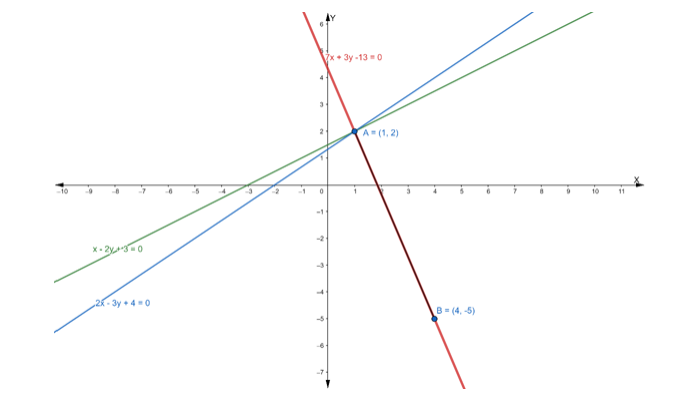# Find the equation of the line drawn through the point of intersection of theQuestion:

Find the equation of the line drawn through the point of intersection of the lines x – 2y + 3 = 0 and 2x – 3y + 4 = 0 and passing through the point (4, -5).

Solution:

Suppose the given two lines intersect at a point $P\left(x_{1}, y_{1}\right) .$ Then, $\left(x_{1}, y_{1}\right)$ satisfies each of the given equations.

$x-2 y+3=0 \ldots(\mathrm{i})$

$2 x-3 y+4=0 \ldots(\mathrm{ii})$

Now, we find the point of intersection of eq. (i) and (ii)

Multiply the eq. (i) by 2 , we get

$2 x-4 y+6=0 \ldots$ (iii)

On subtracting eq. (iii) from (ii), we get

$2 x-3 y+4-2 x+4 y-6=0$

$\Rightarrow y-2=0$

$\Rightarrow y=2$

Putting the value of y in eq. (i), we get

$x-2(2)+3=0$

$\Rightarrow x-4+3=0$

$\Rightarrow x-1=0$

$\Rightarrow x=1$

Hence, the point of intersection $\mathrm{P}\left(\mathrm{x}_{1}, \mathrm{y}_{1}\right)$ is $(1,2)$Let $A B$ is the line drawn from the point of intersection $(1,2)$ and passing through the point $(4,-5)$

Firstly, we find the slope of the line joining the points (1, 2) and (4, -5)

Slope of line joining two points $=\frac{\mathrm{y}_{2}-\mathrm{y}_{1}}{\mathrm{x}_{2}-\mathrm{x}_{1}}$

$\therefore \mathrm{m}_{\mathrm{AB}}=\frac{-5-2}{4-1}=\frac{-7}{3}$

Now, we have to find the equation of line passing through point (4, -5)

Equation of line: $y-y_{1}=m\left(x-x_{1}\right)$

$\Rightarrow \mathrm{y}-(-5)=-\frac{7}{3}(\mathrm{x}-4)$

$\Rightarrow \mathrm{y}+5=-\frac{7}{3}(\mathrm{x}-4)$

$\Rightarrow 3 y+15=-7 x+28$

$\Rightarrow 7 x+3 y+15-28=0$

$\Rightarrow 7 x+3 y-13=0$

Hence, the equation of line passing through the point $(4,-5)$ is $7 x+3 y-13=0$